### Mathematics SSS1 Third Term Final Assessment

In the shapes below, calculate each of the angles marked

1.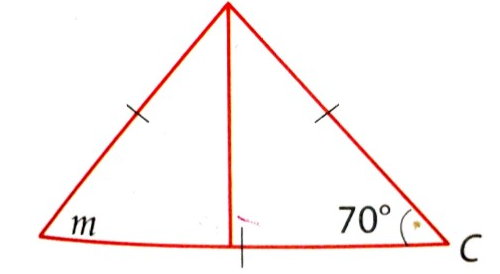1. m = 70
2. m = 75
3. m = 80
4. m = 85

2.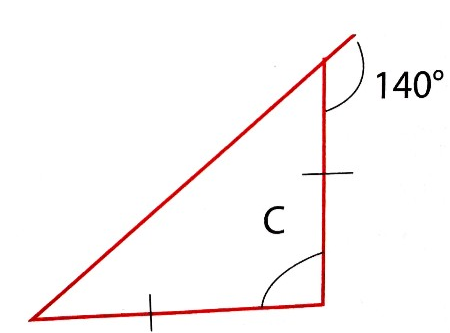1. c = 100
2. c = 150
3. c = 140
4. c = 130

3.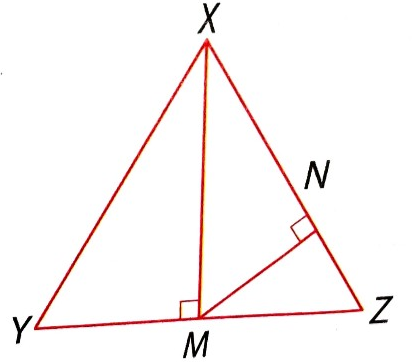1. NMX = 60
2. NMX = 64
3. NMX = 65
4. NMX = 55

4.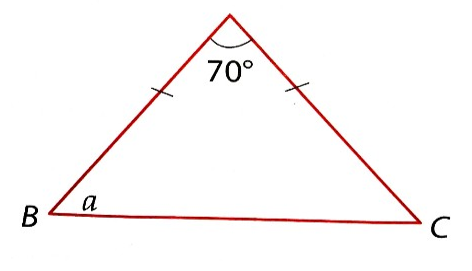1. a = 55
2. a = 56
3. a = 57
4. a = 58

5.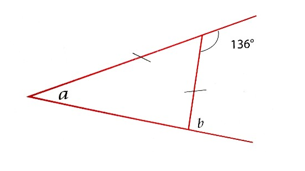1. a = 65
2. View More